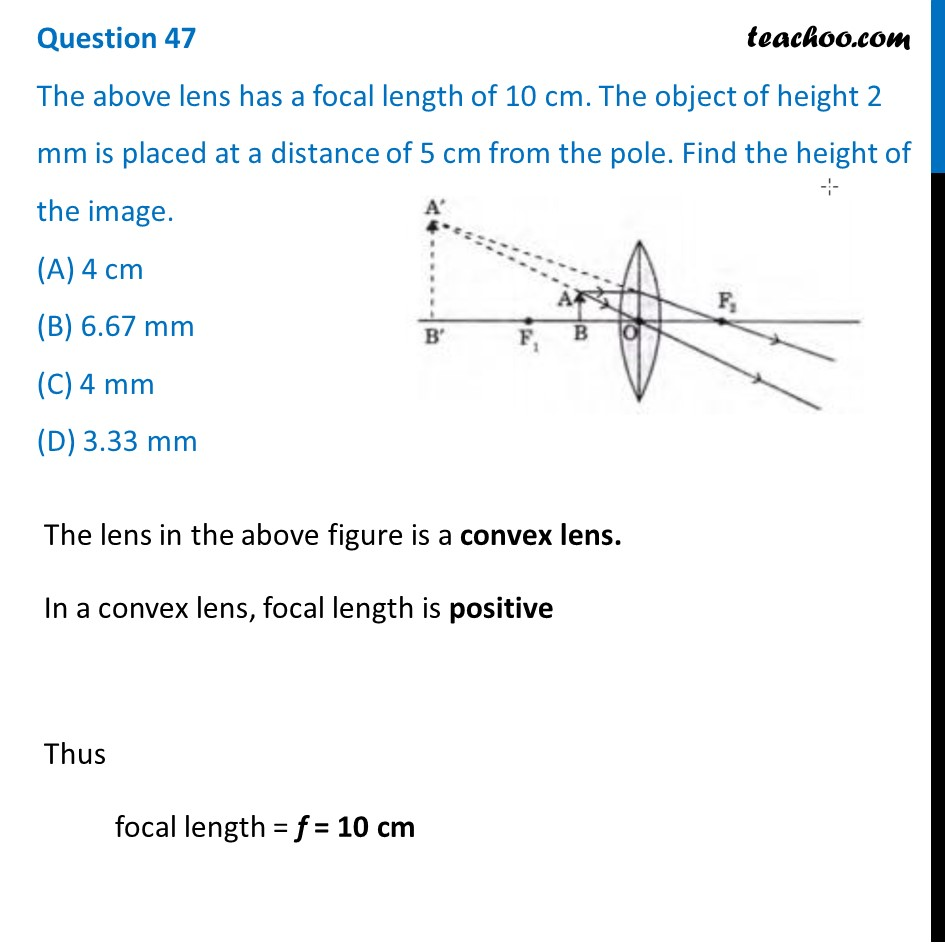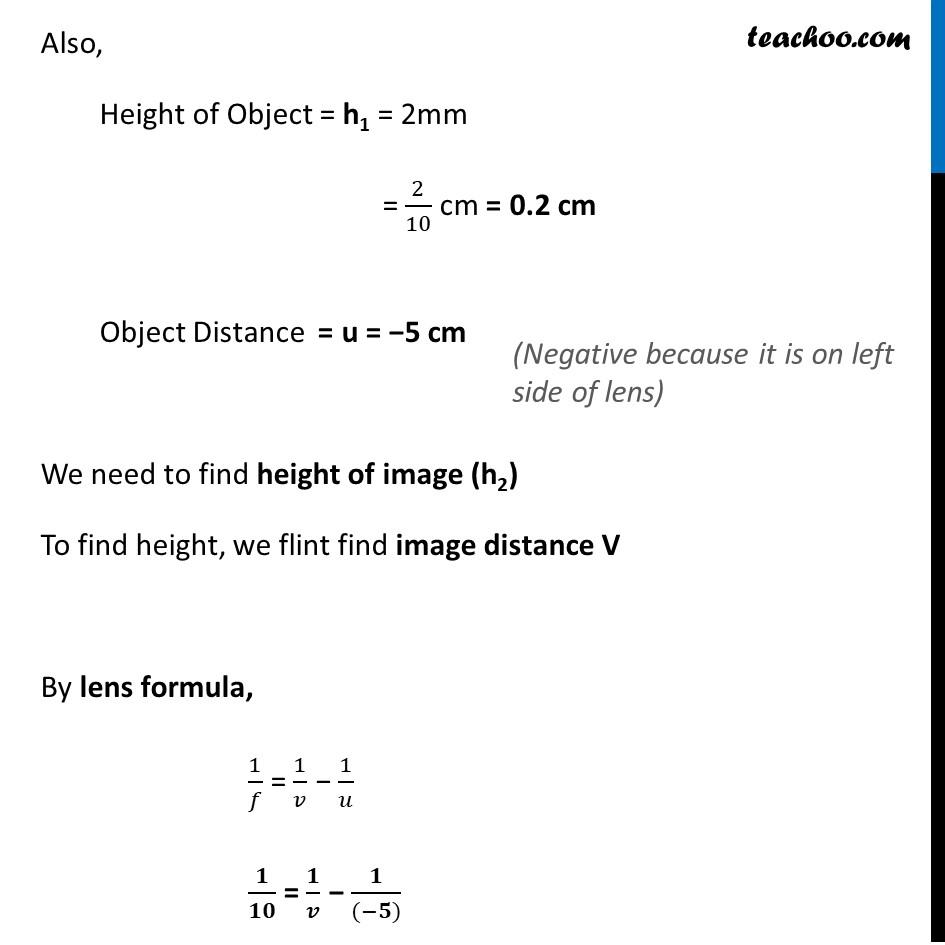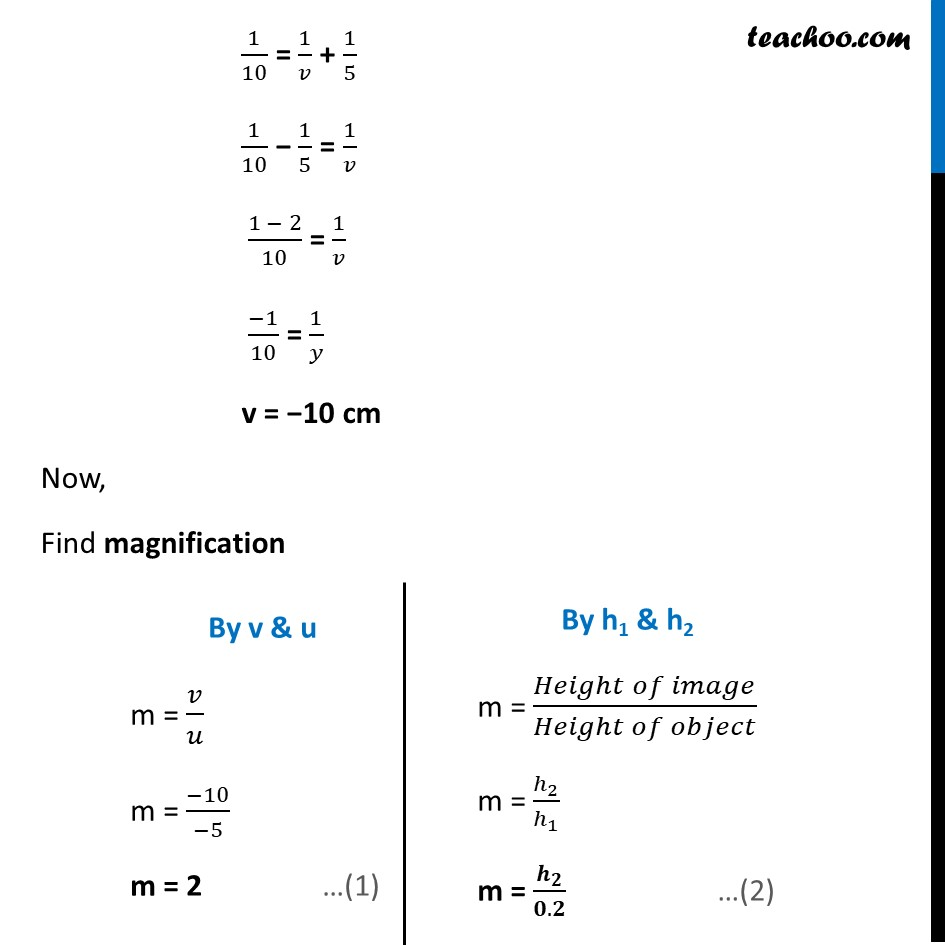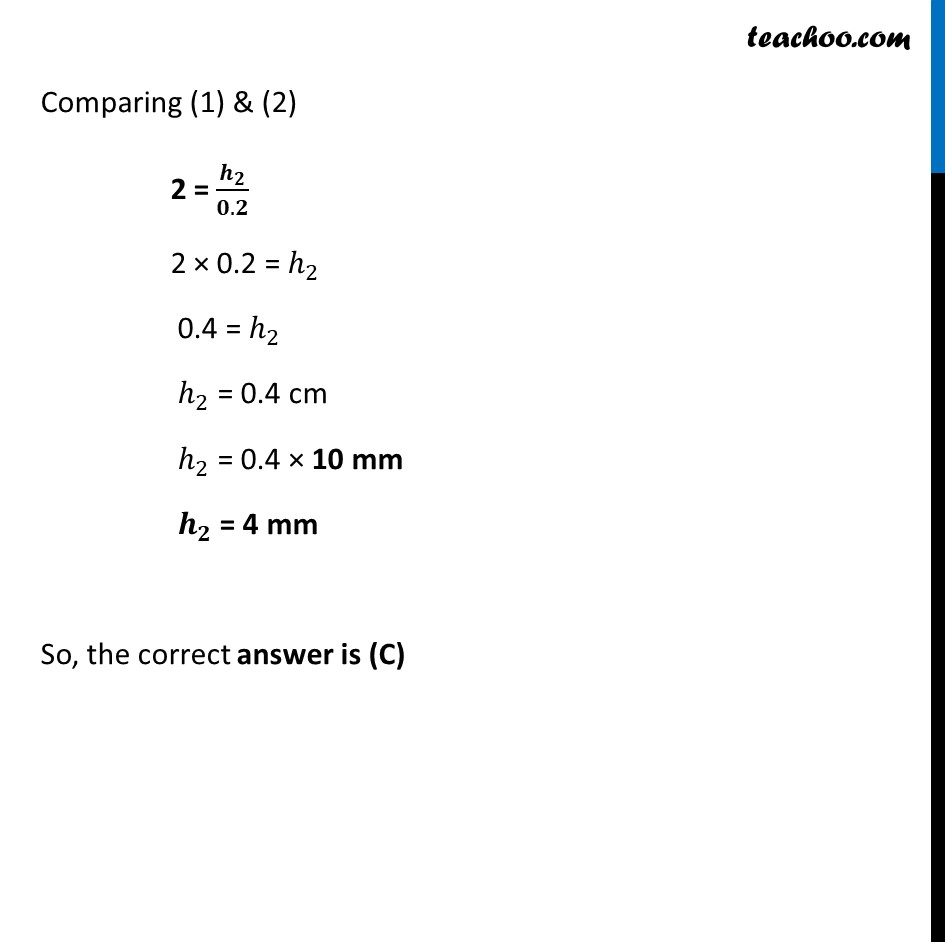Solutions - CBSE Class 10 Sample Paper for 2022 Boards - Science [MCQ]

Class 10
Solutions to CBSE Sample Paper - Science Class 10

## (D) 3.33 mmLearn in your speed, with individual attention - Teachoo Maths 1-on-1 Class

### Transcript

Question 47 The above lens has a focal length of 10 cm. The object of height 2 mm is placed at a distance of 5 cm from the pole. Find the height of the image. (A) 4 cm (B) 6.67 mm (C) 4 mm (D) 3.33 mm The lens in the above figure is a convex lens. In a convex lens, focal length is positive Thus focal length = f = 10 cm Also, Height of Object = h1 = 2mm = 2/10 cm = 0.2 cm Object Distance = u = −5 cm We need to find height of image (h2) To find height, we flint find image distance V By lens formula, 1/𝑓 = 1/𝑣 − 1/𝑢 𝟏/𝟏𝟎 = 𝟏/𝒗 − 𝟏/((−𝟓)) 1/10 = 1/𝑣 + 1/5 (Negative because it is on left side of lens) 1/10 = 1/𝑣 + 1/5 1/10 − 1/5 = 1/𝑣 (1 − 2)/10 = 1/𝑣 (−1)/10 = 1/𝑦 v = −10 cm Now, Find magnification By v & u m = 𝑣/𝑢 m = (−10)/(−5) m = 2 …(1) By h1 & h2 m = (𝐻𝑒𝑖𝑔ℎ𝑡 𝑜𝑓 𝑖𝑚𝑎𝑔𝑒)/(𝐻𝑒𝑖𝑔ℎ𝑡 𝑜𝑓 𝑜𝑏𝑗𝑒𝑐𝑡) m = ℎ_2/ℎ_1 m = 𝒉_𝟐/(𝟎.𝟐) …(2) Comparing (1) & (2) = 2 = 𝒉_𝟐/(𝟎.𝟐) = 2 × 0.2 = ℎ_2 = 0.4 = ℎ_2 ℎ_2 = 0.4 cm ℎ_2 = 0.4 × 10 mm 𝒉_𝟐 = 4mm So, the correct answer is (C)